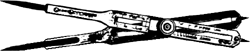# proportional dividers

## proportional dividers

[prə′pȯr·shən·əl di′vīd·ərz]
(design engineering)
Dividers with two legs, pointed at both ends, and an adjustable pivot; distances measured by the points at one end can be marked off in proportion by the points at the other end.

## proportional dividersproportional dividers
A drafting instrument used in reducing or enlarging drawings; consists of two legs whose ends are pointed and cross each other (like the letter X) at a pivot point whose position can be adjusted; the distance between the two pointed ends on one side of the pivot is proportional to the distance between the pointed ends on the opposite side.
Site: Follow: Share:
Open / Close